## provides one model for solving this problem. The maximum strength of the earth’s magnetic field is about 6.9 x 10-5 T near the south magneti

Question

provides one model for solving this problem. The maximum strength of the earth’s magnetic field is about 6.9 x 10-5 T near the south magnetic pole. Suppose we want to utilize this field with a rotating coil to generate 84.5-Hz ac electricity What is the minimum number of turns (area per turn = 0.021 m2) that the coil must have to produce an rms voltage of 170 V?

in progress 0
1 month 2021-08-05T10:28:55+00:00 1 Answers 2 views 0

The minimum no. of turns is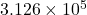Explanation:

Given:

Magnetic field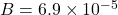T

Frequency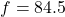Hz

Area of turn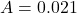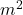Voltage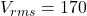V

From the formula of induced emf,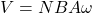Where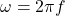and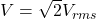So number of turn is,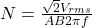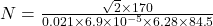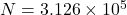Therefore, the minimum no. of turns is